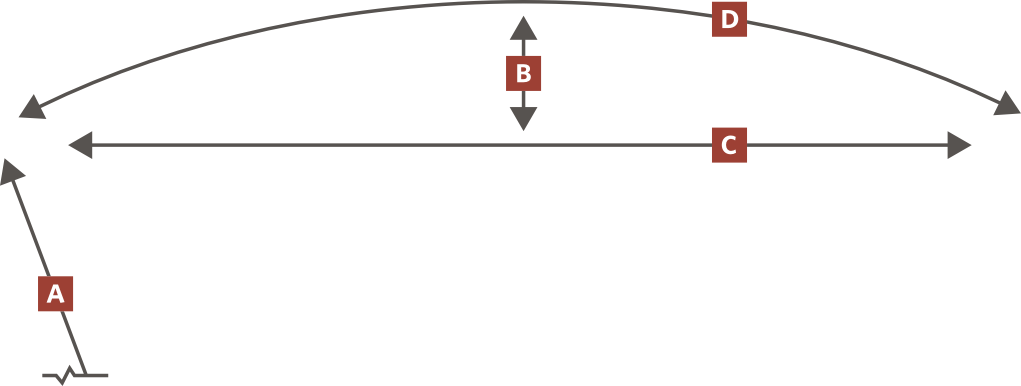#Easy as 1,2,3
• CHOOSE YOUR MATERIAL AND UNIT OF MEASUREMENT
• ENTER THE RADIUS [A] OR HEIGHT [B]
• ENTER THE CHORD [C] OR ARC [D] LENGTH
1

### CHOOSE YOUR MATERIAL AND UNIT OF MEASUREMENT

 Polymer Cast Iron Galvanized Steel 16ga10ga Feet Metric

2

3

### ENTER THE CHORD OR ARC LENGTH

Have Chord Length Have Arc Length
 Chord Length: feet

Estimate the amount of alternating tiles and wedges needed to cover area. Example: A 15R ramp 12' wide would need roughly 5 - 15R wedges and 6 - 2' x 2' Tiles (6x2=12)
8.8R=15R+2' x 1'
11.5R=20R+2' x 1'
14.1R=25R+2' x 1'
15R=15R+2' x 2'
16.8R=30R+2' x 1'
18R=15R+2' x 2.5' *
*cast iron only
20R=20R+2' x 2'
21R=15R+2' x 3'
24.2R=20R+2' x 2.5' *
*cast iron only
25R=25R+2' x 2'
27.2R=15R+2' x 4'
28.5R=20R+2' x 3'
30R=30R+2' x 2'
30.4R=25R+2' x 2.5' *
*cast iron only
33.4R=15R+2' x 5'
35.8R=25R+2' x 3'
36.6R=30R+2' x 2.5' *
*cast iron only
37R=20R+2' x 4'
43.3R=30R+2' x 3'
45.5R=20R+2' x 5'
46.7R=25R+2' x 4'
56.5R=30R+2' x 4'
57.5R=25R+2' x 5'
69.8R=30R+2' x 5'

### USING EASYARC

Select unit of measure and material. Enter R-value and Chord length then click the 'Calculate' button to create the estimate for your radius installation. (If R-value is unknown, enter the chord length and height. Arc length may be used as an alternative to the chord in previous step.) The recommended tile size, wedge size and their respective quantities are displayed.

The values ‘arc length’, ‘segment length’, ‘arc overrun’ and 'variance' are informational only.

Arc length is calculated when the chord length is given. Segment length is known by adding the top edge lengths of 1 selected tile and wedge. Arc overrun is the remainder when the arc length is different than the sum of the tiles and wedges. Variance occurs when the radius calculated is different than the radius made from the suggested components. The variance amount is the distance between those two values.

For additional assistance contact customer service at 1-888-860-8897.